## Example Questions

### Example Question #1 : How To Find Consecutive Integers

If four consecutive odd integers greater than 9 are added together, what is the smallest possible sum of those four integers?Explanation:

The 4 consecutive of integers greater than 9 (but not including 9) are 11, 13, 15, 17.  Added together, we get 56.

### Example Question #2 : How To Find Consecutive Integers

There are two consectutive positive integersand, and their product is 132.

What is the value of the larger integer?Explanation:

to find the integers you can guess and check (you know both are larger than 10 because their product is greater than 100) or you can set up a system of equations.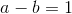if a is the larger number and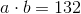.

Therefore: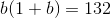if you solve that quadratic you get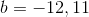and b is the smaller number so the bigger number is 12

### Example Question #3 : How To Find Consecutive Integers

Five students are lined up by height so that their heights are ordered in a consecutive manner. If the sum of their heights (in inches) is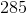inches, what is the height of the second tallest student?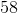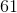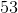Explanation:

For a problem like this, you can always use the answers to find your correct answer. By choosing each number, you can find the other two options and then add together your values. You would, for instance, takeand say, "The list must be: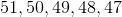." Then, adding them to get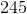, you will know that this is not correct.

However, you can do this much more easily with algebra. You know that five consecutive integers are going to look like: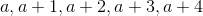, whereis the height of the shortest person. Thus, you know that the total inches of the students can be represented in the following manner: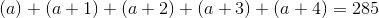This simplifies to: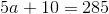Solving for, you get: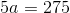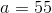However, remember that you need to find the second tallest person. This means that your list is: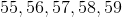. Thus, your answer is.

### Example Question #4 : How To Find Consecutive Integers

The sum of the squares of three consecutive odd integers is.

Which of the following is the smallest of of these three numbers?Not able to be determined.Not able to be determined.

Explanation:

An odd integer can be expressed as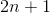because two times any number is an even number and one plus an even number is always odd. We can then write these three consecutive odd integers in terms ofas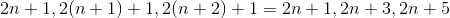. We can then square each of these numbers and add them together.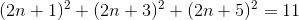Then use binomial expansion to rewrite the expression (better known as FOIL).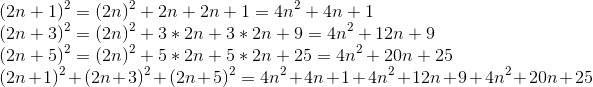We can then combine like terms and set it equal toas given.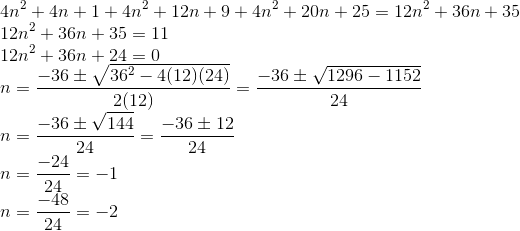This tells us that two possible sets of numbers satisfy this condition: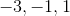and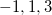. It is evident that the sums of the squares of these numbers should be the same, so we cannot determine which set the question is discussing.

### Example Question #5 : How To Find Consecutive Integers

What is the next number in the geometric sequence?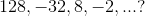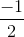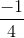Explanation:

A geometric sequence is one where two get two each consecutive number in the sequence, you must multiply or divide a number.  If we look at the sequence, we can see that the pattern is dividing byeach time.  Therefore, to get the next term in the sequene, we must divide the last term given in the sequence: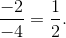### Example Question #6 : How To Find Consecutive Integers

The prices of three candies are consecutively priced. If the total price of the candies is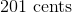, what is the cost of the highest priced candy?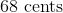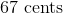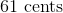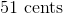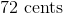Explanation:

For a problem like this, you can always use the answers to find your correct answer. By choosing each number, you can find the other two options and then add together your values. You would, for instance, take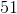and say, "The other two must beand." Then, adding them to get, you will know that this is not correct.

However, you can do this much more easily with algebra. You know that three consecutive integers are going to look like: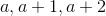, whereis the price of the least expensive candy. Thus, you know that the total price of your candies can be represented in the following manner: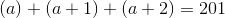This simplifies to: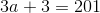Solving for, you get: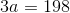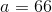Remember that you need to find the highest priced candy. Therefore, the answer is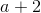or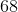.

### Example Question #7 : How To Find Consecutive Integers

256, 64, 16, 4 ... ?

The common ratio of consecutive terms is 4.

The seventh term is.

The common difference of consecutive terms is 4.

The sum of the fourth and fifth terms is 20.

The fifth term is 2.

The seventh term is.

Explanation:

In this geometric sequence, the numbers get smaller; therefore, there will be a fractional ratio. We can determine the common ratio by dividing one of the terms by the term immediately preceding it. In the above sequence, use the smallest numbers, 4 and 16, in order to make the calculation the easiest.Now, address which four of the five statements are false.

There is a common ratio, not a common difference, in a geometric sequence. Thus the answer choice that mentions "common difference" is incorrect.

The common ratio is, not 4. Thus, the common ratio answer choice is incorrect.

The fifth term is 1 in this sequence, not 2, which eliminates another answer choice.

Finally, the sum of the third and fourth terms is 20, and the sum of the fourth and fifth terms is 5; the only possible correct answer is "The seventh term is."